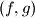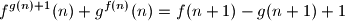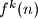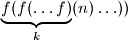### IMO Shortlist 2011 problem A4

Kvaliteta:
Avg: 5,0
Težina:
Avg: 7,0
Determine all pairs$(f,g)$ of functions from the set of positive integers to itself that satisfyfor every positive integer$n$. Here,$f^k(n)$ means$\underbrace{f(f(\ldots f)}_{k}(n) \ldots ))$.

Proposed by Bojan Bašić, Serbia
Izvor: Međunarodna matematička olimpijada, shortlist 2011